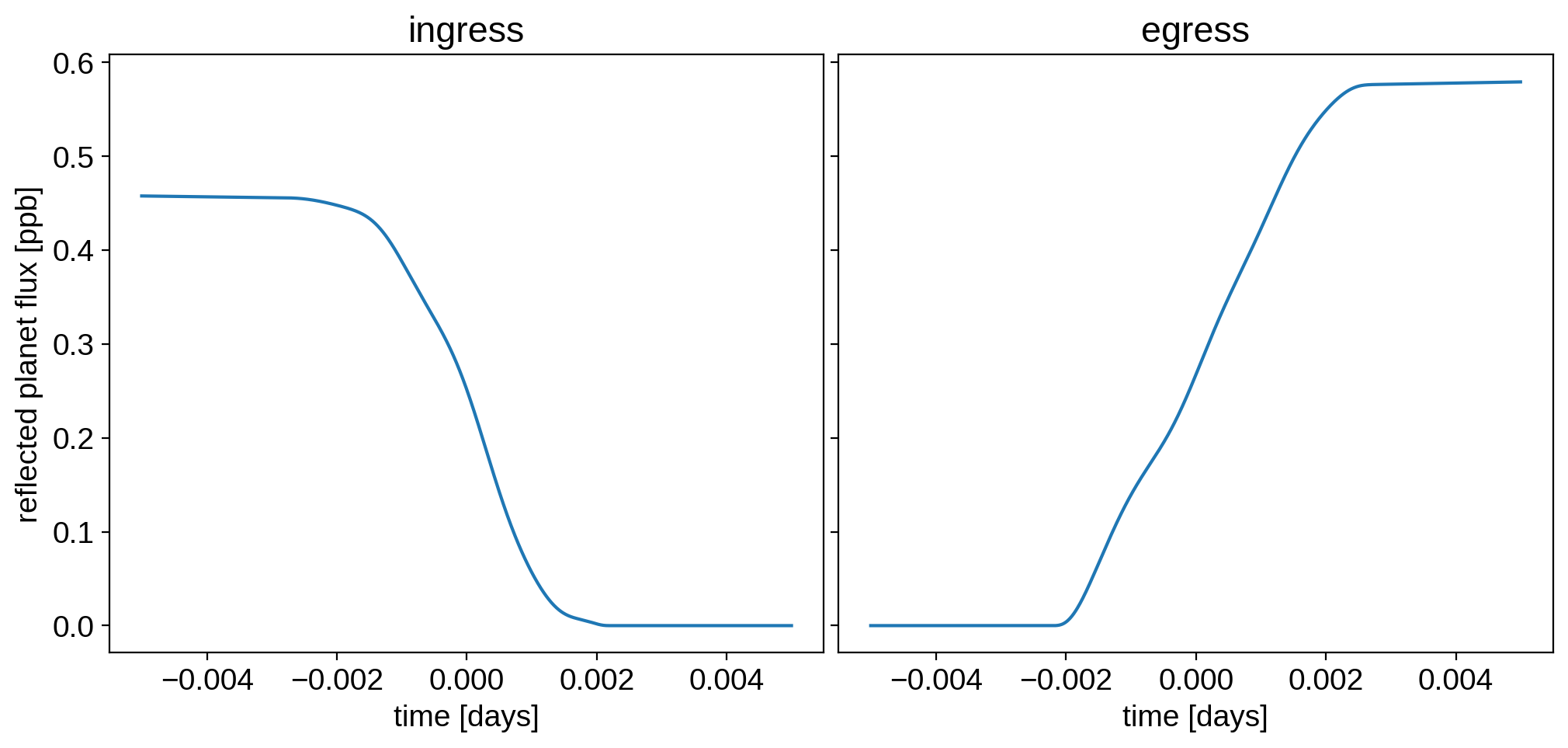Note

This tutorial was generated from a Jupyter notebook that can be downloaded here.

# Maps in reflected light¶

This notebook discusses how to model phase curves and occultation light curves in reflected light. The paper describing the theory, the algorithm, and the implementation lives here: https://github.com/rodluger/starrynight.

## Instantiating a reflected light map¶

Let’s begin by instantiating a map in reflected light. We do this by specifying reflected=True when calling starry.Map().

:

import matplotlib.pyplot as plt
from matplotlib import colors
import numpy as np
import astropy.units as u
import starry

starry.config.lazy = False
starry.config.quiet = True

:

map = starry.Map(ydeg=15, reflected=True)


Before we set any spherical harmonic coefficients, let’s take a look at our map. We can call the show() method as usual:

:

map.show()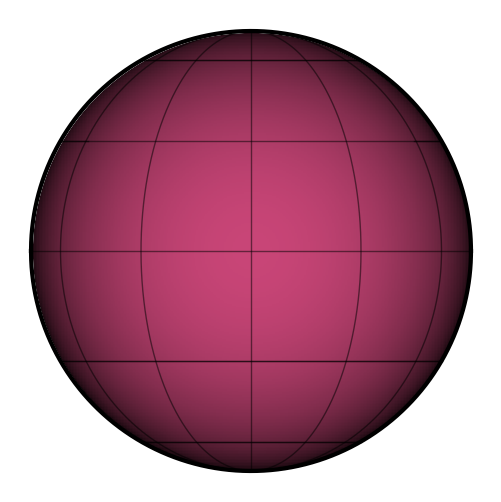By default, the illumination source is along the $$+\hat{z}$$ direction at $$z = 1$$, so directly in front of the object, one unit away. In this case, the starry normalization results in a sub-illumination intensity of $$1/\pi$$, a value that falls off as the cosine of the viewing angle to zero at the limb. (As we’ll see below, this results in the integral of the illumination over the disk to be equal to $$2/3$$, the geometric albedo of a Lambert sphere.)

Looking at the figure above, you can easily tell that points in the center of the map (where it is noon) are brighter than points along the edges (where it is dawn or dusk). To change the location of the illumination source, we edit the xs, ys, and zs keywords, just as we do when calling the flux() method. These are the Cartesian coordinates of the illumination source.

:

map.show(xs=1, ys=0, zs=0)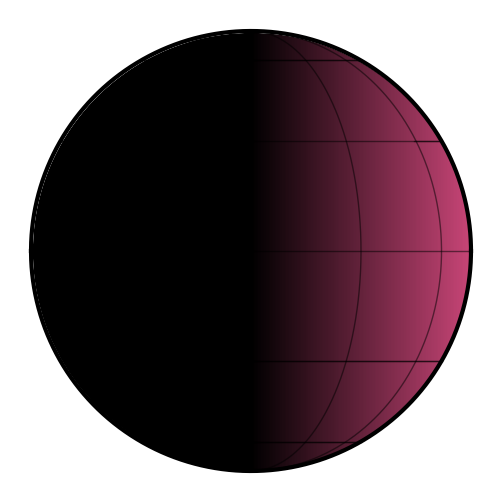We are now viewing a uniform map illuminated from the side. The intensity on the left half is zero, since it is completely unilluminated.

By the way, we can see the albedo map of the body by specifying illuminate=False in the call to show:

:

norm = colors.Normalize(vmin=0, vmax=1.25)
map.show(illuminate=False, colorbar=True, norm=norm)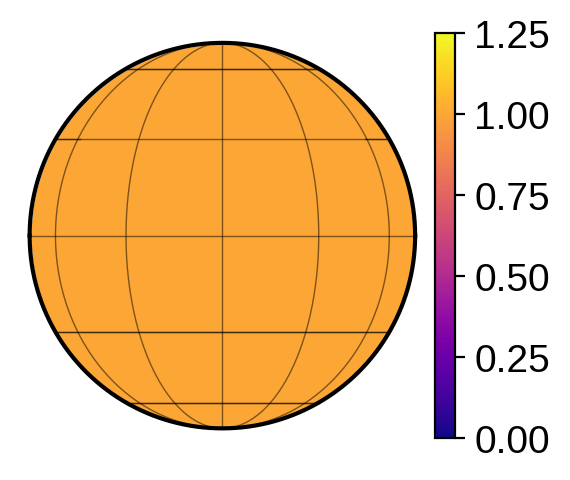By default, the albedo is unity everywhere. If all we wish to change is the average albedo of the planet, we do this via the amplitude map.amp parameter:

:

map.amp = 0.75

:

map.show(illuminate=False, colorbar=True, norm=norm)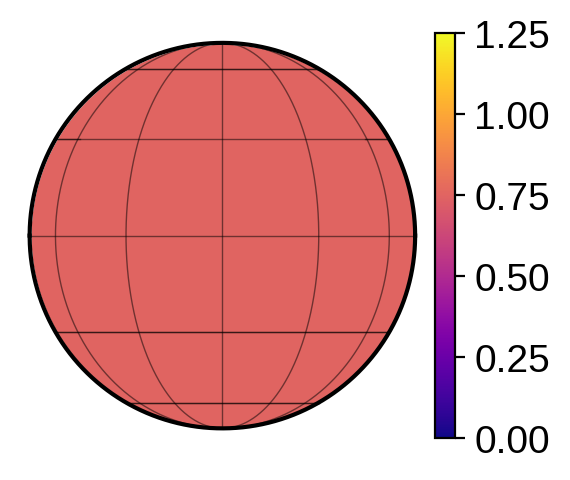The amplitude parameter corresponds to the average spherical albedo of the body (a quantity closely related to the Bond albedo, but at a single wavelength).

## Normalization and units¶

The distance between the body and the source in units of the body’s radius, $$r_s = \sqrt{x_s^2 + y_s^2 + z_s^2}$$, controls the overall amplitude of the intensity on the surface and the total flux from the body. We can check that it follows the expected one-over-r-squared law:

:

map.amp = 1.0
r = np.logspace(0, 2)
plt.figure(figsize=(12, 5))
plt.plot(r, map.intensity(lat=0, lon=0, xs=0, ys=0, zs=r).reshape(-1), label="measured")
plt.plot(r, 1 / (np.pi * r ** 2), label=r"$I(r) = \frac{1}{\pi r_s^2}$", ls="--")
plt.plot(1, 1 / np.pi, "ko")
plt.axvline(1, color="k", ls="--", lw=1, alpha=0.5)
plt.axhline(1 / np.pi, color="k", ls="--", lw=1, alpha=0.5)
plt.yscale("log")
plt.xscale("log")
plt.legend(fontsize=18)
plt.xlabel("star-planet distance", fontsize=24)
plt.ylabel("substellar intensity", fontsize=24);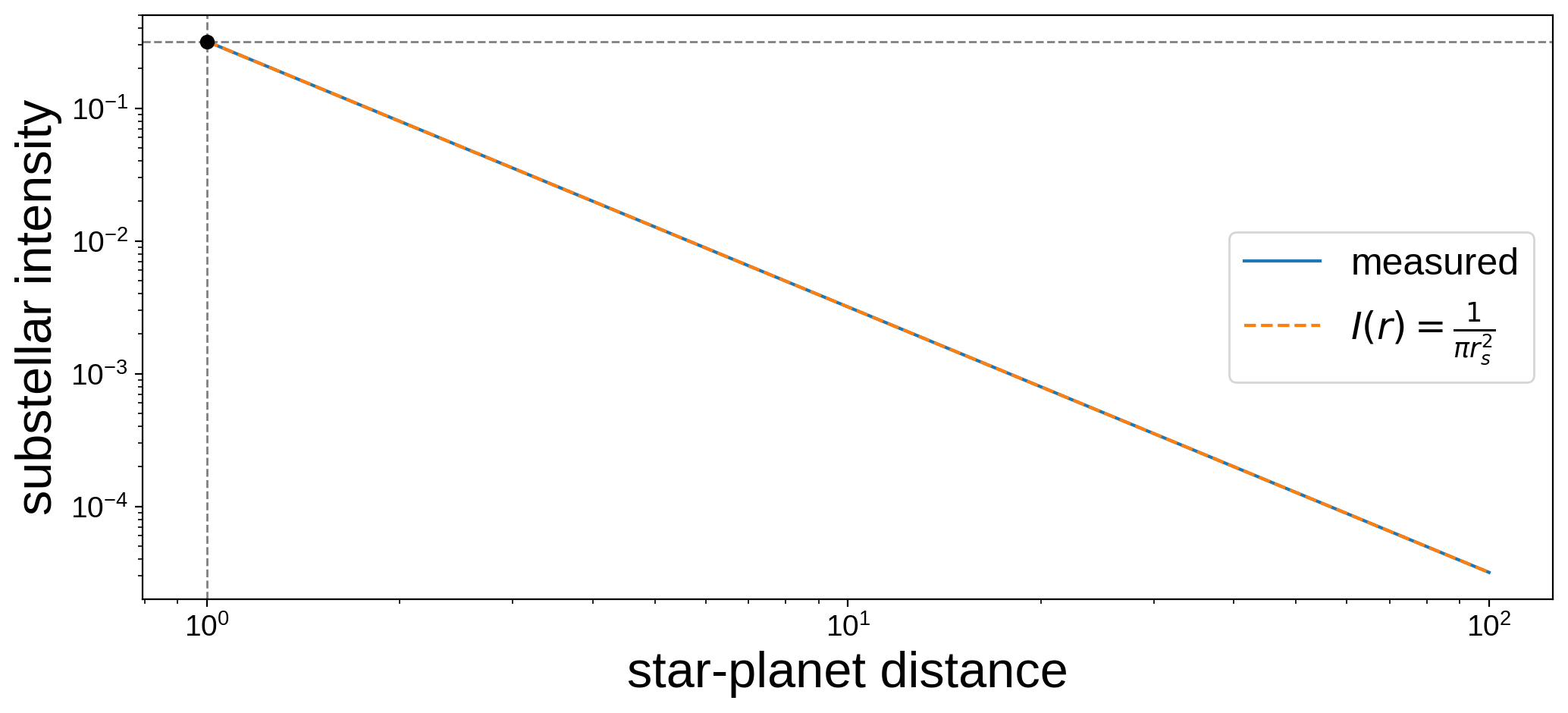In particular, the reflected intensity (map.intensity) of a uniform body at a point on the surface an angle $$\phi$$ away from the sub-stellar point is given by

$I(\phi) = \frac{A}{\pi r_s^2} \, \mathrm{max}(0, \cos\phi)$

where $$A$$ is the body’s (spherical) albedo, which we expand in terms of spherical harmonics. This quantity is unitless, and that factor of $$\pi$$ ensures the proper normalization for a Lambert sphere (see below).

Now, the flux (map.flux) measured from the source is the surface integral of the intensity over the visible portion of the sky-projected disk, so it also scales in the same way. For reference, let’s compute the phase curve of a uniform, unit-albedo body at unit distance from the illumination source, seen edge-on over a full year. Here’s a movie of what we’re computing:

:

theta = np.linspace(0, 2 * np.pi, 50)
map.show(xs=np.sin(theta), ys=0, zs=np.cos(theta))


And here’s the corresponding light curve:

:

plt.figure(figsize=(12, 5))
phase = np.linspace(0, 1, 1000)
theta = np.linspace(0, 2 * np.pi, 1000)
plt.plot(phase, map.flux(xs=np.sin(theta), ys=0, zs=np.cos(theta)))
plt.axhline(0, color="C3", ls="--")
plt.axhline(2 / 3, color="C3", ls="--")
plt.xlabel("orbital phase")
plt.ylabel("reflected planet flux");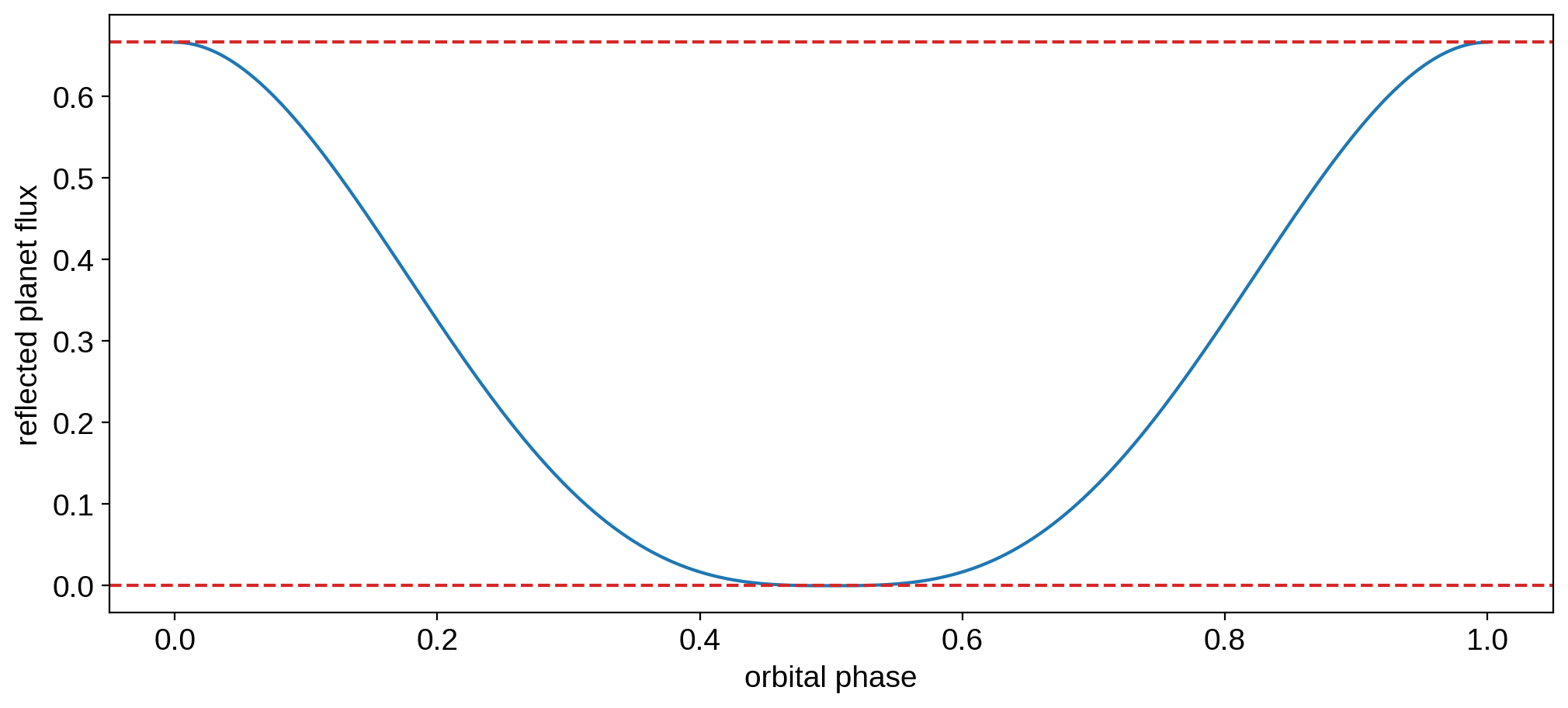Note, in particular, the minimum and maximum values (dashed red lines). When the planet is in a transiting configuration (phase = 0.5), the total flux is zero, since only the nightside is visible. When the planet is at secondary eclipse, the intensity convention described above means that the total flux returned by starry is $$2/3$$. This value is precisely the geometric albedo of a Lambertian reflector whose spherical albedo is unity (see, e.g., Section 4.5 of Schwartz & Cowan 2015).

## Setting the albedo distribution¶

Moving on, reflected light maps behave in a similar way as regular spherical harmonic maps, except the spherical harmonic coefficients y represent the expansion of the surface albedo distribution rather than the emissivity distribution. Let’s load the continental map of the Earth and look at the albedo distribution:

:

map.load("earth", sigma=0.075)

:

map.show(projection="moll", illuminate=False, res=500, colorbar=True)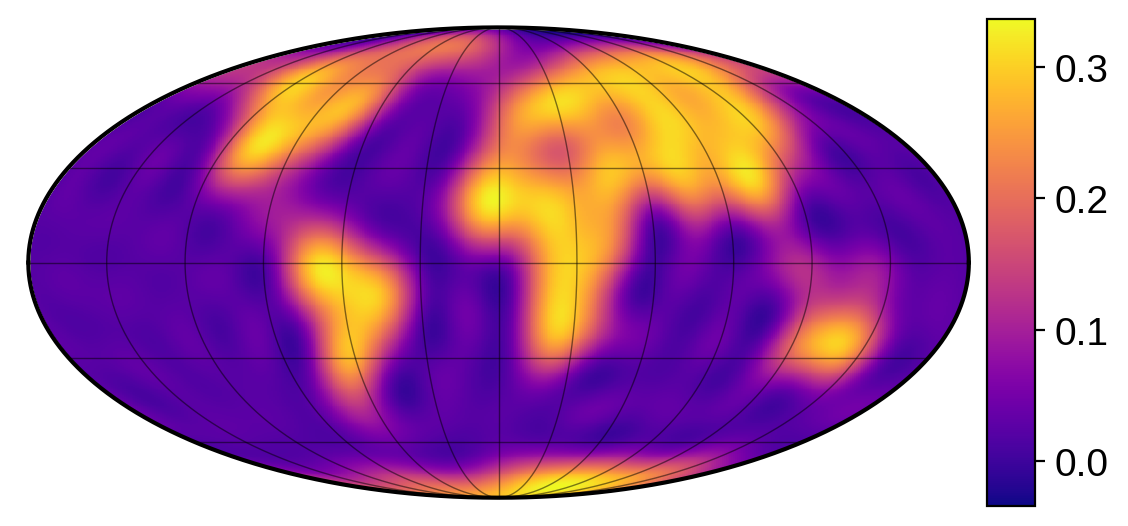The image we loaded is a grayscale image with unit dynamic range: the oceans have zero albedo and land has unit albedo. This isn’t true of the real Earth, whose continents have an albedo closer to 0.4 on average (although the exact value depends on wavelength).

To fix this, we can scale the map amplitude:

:

map.amp *= 0.4


Note

By default, when you instantiate a map, the map amplitude (amp) is set to unity. But when you load a map from an image, starry needs to adjust amp to get the dynamic range of the spherical harmonic expansion to match that of the input image, so amp will in general be different from unity. For that reason, we do map.amp *= 0.4 instead of setting it to 0.4 directly, since (as you can check) the map amplitude is not equal to one when you load the image of the Earth.

Here’s the new albedo distribution, which is more realistic (although we’re still assuming a cloudless planet):

:

map.show(projection="moll", illuminate=False, res=500, colorbar=True)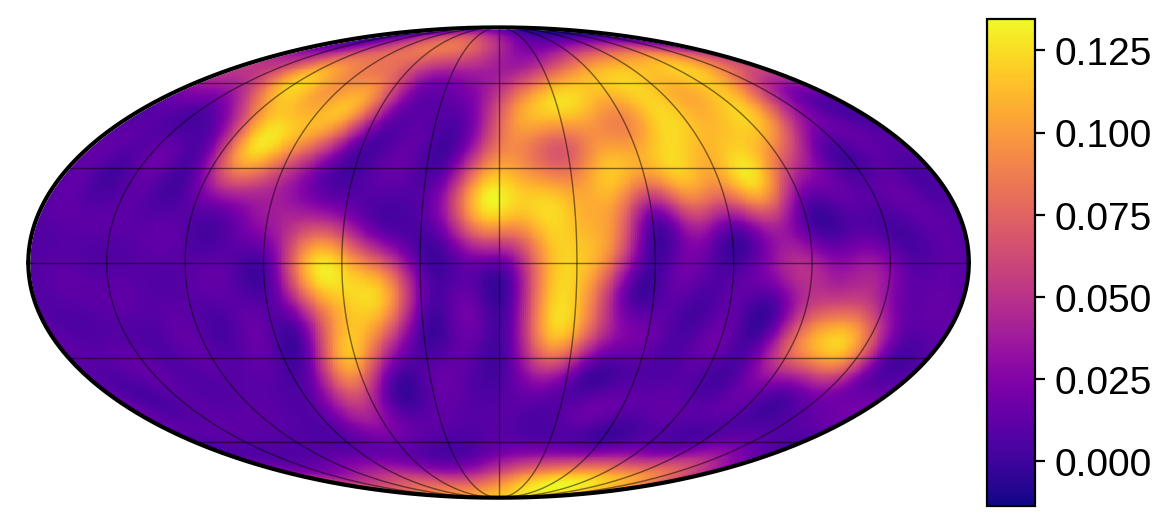Now let’s give the map the same obliquity as the Earth…

:

map.obl = 23.5


… and view the half-Earth rotating over one cycle:

:

map.show(theta=np.linspace(0, 360, 50), xs=1, ys=0, zs=0)


The above animation corresponds to the (northern) winter solstice. Here’s the phase curve of the Earth over one rotation at 8 different illumination phases:

:

fig = plt.figure(figsize=(12, 8))
theta = np.linspace(0, 360, 1000)
phis = np.linspace(0, 360, 9)[:-1]
xs = np.cos((phis - 90) * np.pi / 180)
zs = -np.sin((phis - 90) * np.pi / 180)
for n, phi in enumerate(phis):
plt.plot(theta, map.flux(theta=theta, xs=xs[n], ys=0, zs=zs[n]), label=phi)
plt.xlim(0, 360)
plt.ylim(-0.01, 0.13)
plt.xlabel(r"$\theta$ [degrees]", fontsize=24)
plt.ylabel("Flux", fontsize=24)
legend = plt.legend(
loc="center left", bbox_to_anchor=(1, 0.5), fontsize=36, frameon=False
)
for text in legend.get_texts():
text.set_color("w")
cmap = plt.get_cmap("plasma")
cmap.set_under("#000000")
for n in range(8):
ax = fig.add_axes((1.05, 0.78 - 0.092 * n, 0.05, 0.075))
map.show(res=300, xs=xs[n], ys=0, zs=zs[n], grid=False, ax=ax)
plt.suptitle("Light curves at different illumination phases", fontsize=24);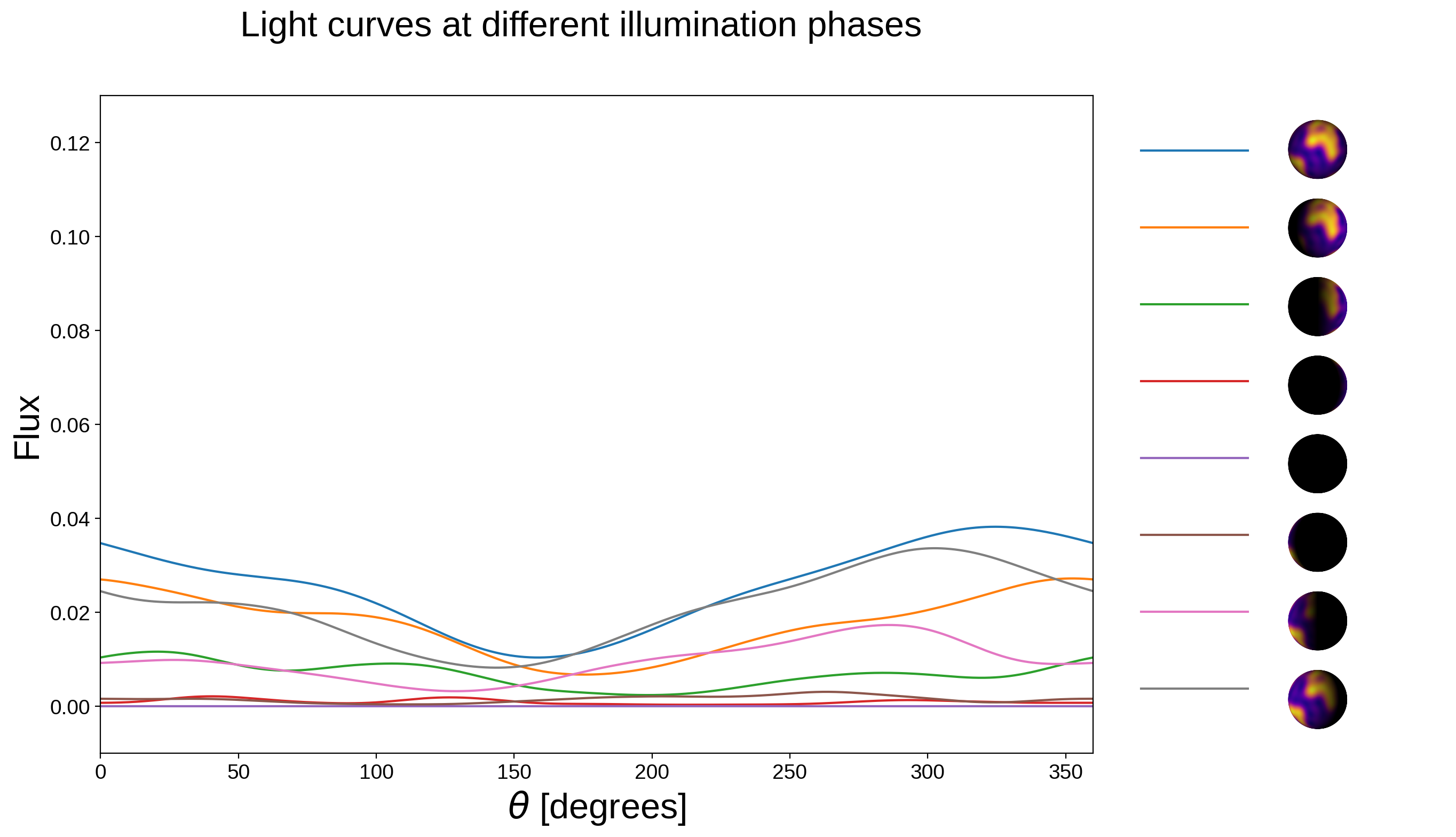## Extended illumination source¶

By default, the illumination source is assumed to be a point source. This is in general fine for most exoplanets, but isn’t a great assumption for close-in planets. In starry, we can account for the finite size of the illumination source by passing the source_npts keyword when instantiating a starry.Map object:

:

map_point_source = starry.Map(reflected=True, source_npts=1)


This quantity corresponds to the number of points used to model the illumination source (i.e., the star). The default is one (a point source). If we increase this number, starry will compute the illumination profile for source_npts uniformly distributed over the projected disk of the star as seen from the planet, and sum over them to obtain an approximate illumination profile. For long period planets, this doesn’t make much of a difference, since the angular size of the star as seen from the planet is quite small. But for short period planets, the star is so large that parts of the planet beyond the usual location of the terminator ($$\pi/2$$ away from the sub-stellar point) are illuminated by the limb of the star. Usually something like 30-50 points are sufficient, but let’s use 300 points to get a very good approximation to the true illumination profile:

:

map_extended_source = starry.Map(reflected=True, source_npts=300)


Now let’s explore this effect below for Kelt-9b, a super short-period hot Jupiter, using parameters from Wong et al. (2019):

:

fig, ax = plt.subplots(2, 2, figsize=(9, 5))

# Keywords for show. We'll visualize the planet
# at full phase in a Mollweide projection.
# We're using values from Wong et al. (2019) here.
aRs = 3.16
RpRs = 0.08229
norm = colors.Normalize(vmin=0, vmax=3.5e-4)
kwargs = dict(
projection="moll", xs=0, ys=0, zs=aRs / RpRs, res=500, screen=False, norm=norm
)

# Show the two maps. Note that for the extended
# source map, we need to pass in the radius of
# the star (in units of the planet's radius).
map_point_source.show(ax=ax[0, 0], **kwargs)
map_extended_source.show(ax=ax[1, 0], rs=1 / RpRs, **kwargs)

# Let's visualize the same two images, but using
# a binary colormap: dark blue for the night side
# and yellow for the dayside.
norm = colors.Normalize(vmin=1e-8, vmax=1.1e-8)
cmap = plt.get_cmap("plasma")
cmap.set_under(cmap(0.0))
cmap.set_over(cmap(1.0))
kwargs.update(cmap=cmap, norm=norm)
map_point_source.show(ax=ax[0, 1], **kwargs)
map_extended_source.show(ax=ax[1, 1], rs=1 / RpRs, **kwargs)

# Annotate
ax[0, 0].annotate(
"point source",
xy=(0, 0.5),
xycoords="axes fraction",
xytext=(-10, 0),
textcoords="offset points",
ha="right",
va="center",
fontsize=12,
)
ax[1, 0].annotate(
"extended source",
xy=(0, 0.5),
xycoords="axes fraction",
xytext=(-10, 0),
textcoords="offset points",
ha="right",
va="center",
fontsize=12,
);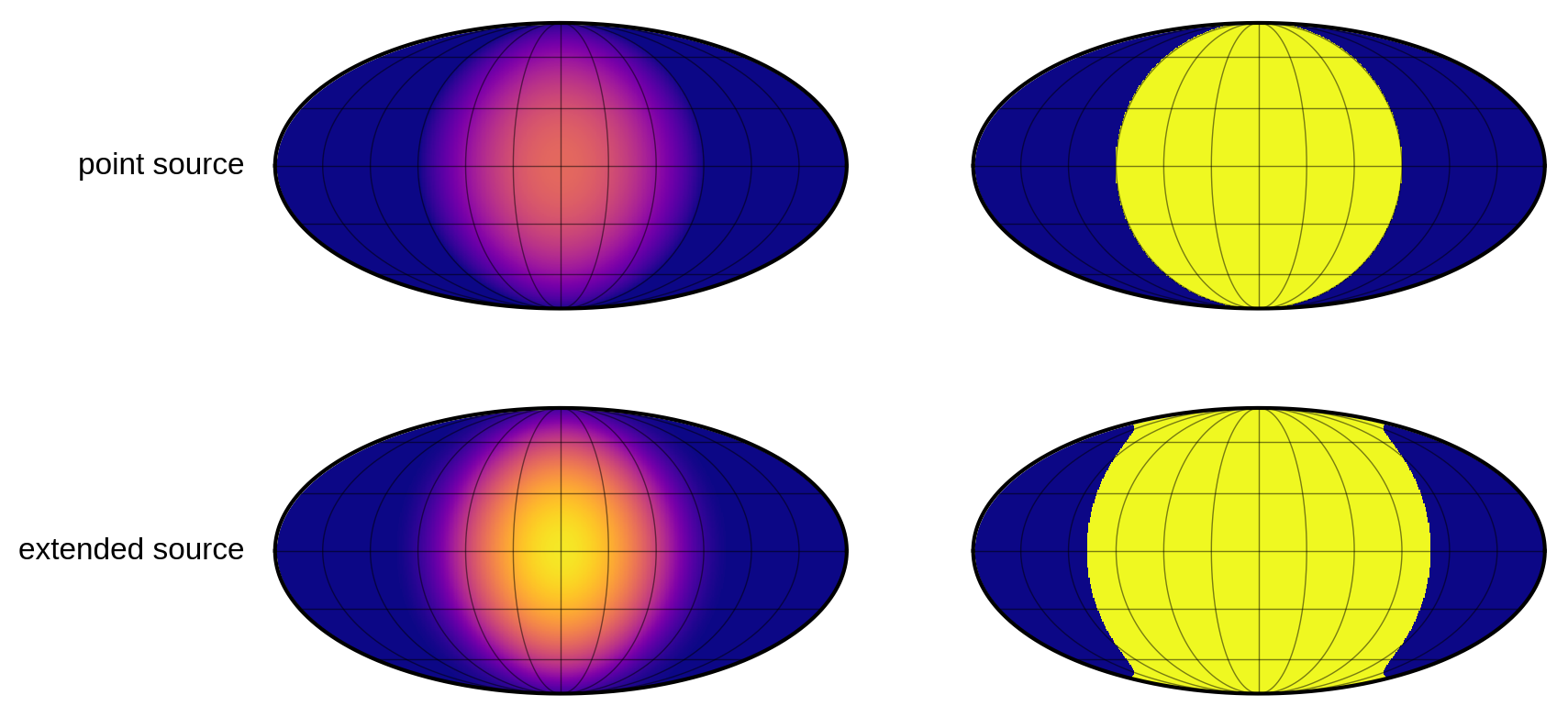The main difference is that the extended source results in an overall larger illumination on the surface. This is due to the simple fact that in the point source case the illumination source is placed at the center of the star, which is one stellar radius farther from the planet than the point closest to the planet (the sub-planetary point) in the extended source case. Once accounting for this difference, the fractional change in the intensity on the planet away from the sub-stellar point is similar in both cases. However, there’s a significant difference near the terminator, which extends about 17$$^\circ$$ degrees farther when we model the extended illumination source (this is much easier to see in the high contrast version at the right).

We can also see how the extended source affects phase curves, assuming a uniform albedo of 0.2 for the planet:

:

map_point_source.amp = 0.2
map_extended_source.amp = 0.2

:

t = np.linspace(0, 1, 1000)
xs = aRs / RpRs * np.sin(2 * np.pi * t)
zs = -aRs / RpRs * np.cos(2 * np.pi * t)
flux_point_source = map_point_source.flux(xs=xs, ys=0, zs=zs)
flux_extended_source = map_extended_source.flux(xs=xs, ys=0, zs=zs, rs=1 / RpRs)
plt.plot(t, 1e6 * flux_point_source, label="point source")
plt.plot(t, 1e6 * flux_extended_source, label="extended source")
plt.legend()
plt.xlabel("phase")
plt.ylabel("flux [ppm]");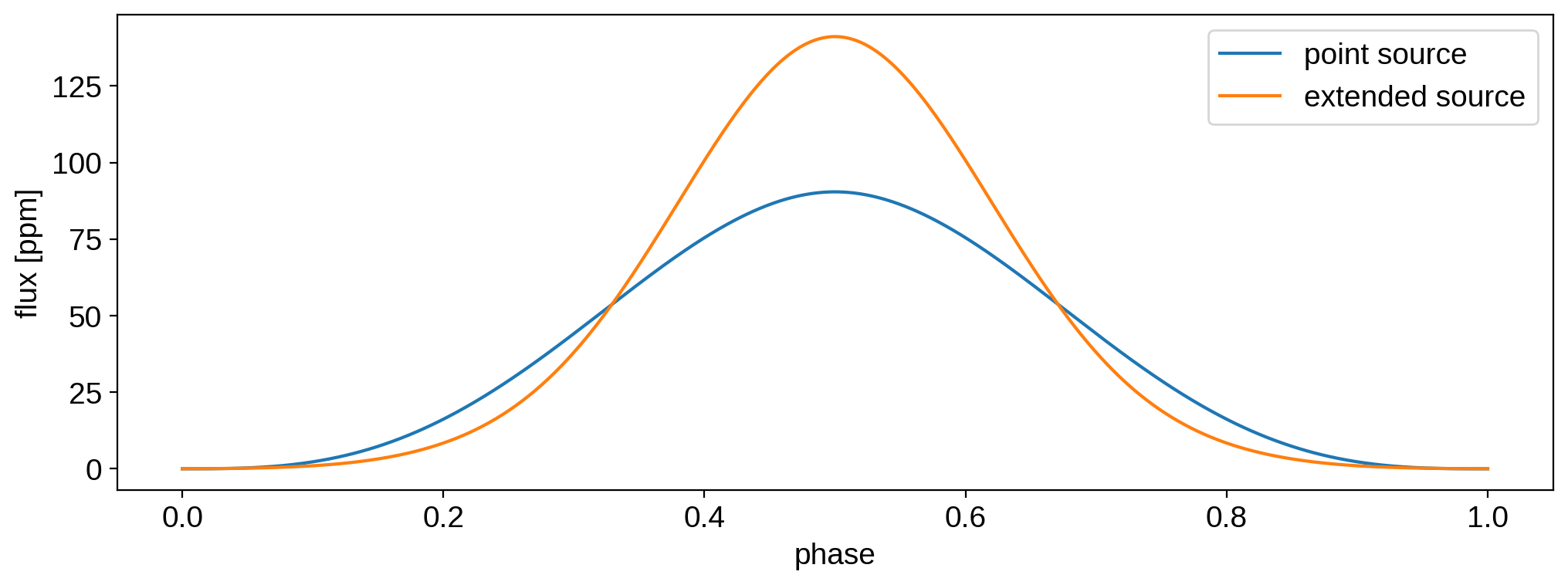The increased illumination at the sub-stellar point results in a $${\sim}30$$ ppm increase in the value of the phase curve close to full phase ($$\pm\frac{1}{2}$$). Close to a phase of zero, the extended source results in decreased flux, since the portion of the star illuminating the planet (the region close to the limb) is slightly farther away, by a factor of $$\sqrt{1 + (\frac{a}{R_\star})^{-2}}$$. This results in a steeper phase curve. Note that we’re not including the transit or the secondary eclipse here (but see below).

## Non-Lambertian scattering¶

The default behavior for reflected light maps in starry is Lambertian scattering, meaning the body reflects light isotropically. This is an idealized case, and real planetary and lunar surfaces don’t usually behave this way. Instead, there is typically a phase dependence to the scattered light. Planets with atmospheres, for instance, preferentially scatter light along the forward and backward direction due to Rayleigh scattering, while rocky bodies with rough surfaces may exhibit a more complex phase dependence.

We’re still working on implementing different scattering laws, but a fairly straightforward model for rough surfaces is that of Oren and Nayar (1994). We implement a simple version of this model in starry, in which the surface roughness is described by a single parameter, $$\sigma$$. The Oren and Nayar model assumes the surface is composed of many tiny facets oriented at random angles, and $$\sigma$$ is equal to the standard deviation of the distribution of their orientations.

In starry, the $$\sigma$$ parameter can be changed by setting the roughness attribute of a map in units of map.angle_unit (degrees, by default). Let’s look at a map with zero surface roughness, i.e., the Lambertian case, over the full range of illumination phases:

:

# Instantiate a map
map = starry.Map(reflected=True)
map.roughness = 0

# View it at all phases
fig, ax = plt.subplots(1, 9)
t = np.linspace(0, 1, 11)[1:-1]
xs = np.sin(2 * np.pi * t)
zs = -np.cos(2 * np.pi * t)
for i in range(9):
map.show(ax=ax[i], xs=xs[i], zs=zs[i], grid=False)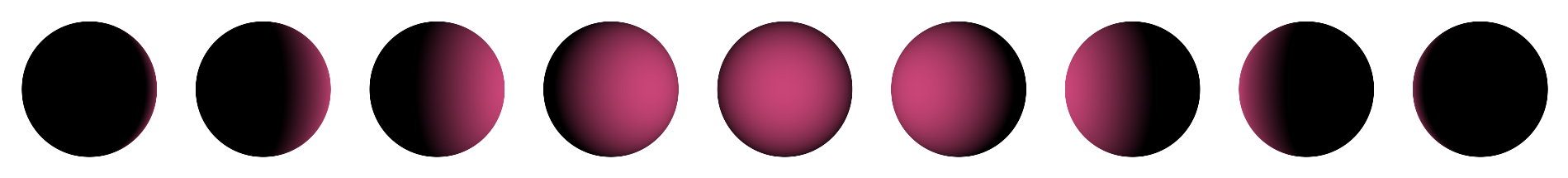Now here’s a map with a very rough surface ($$\sigma = 30^\circ$$):

:

map.roughness = 30
fig, ax = plt.subplots(1, 9)
phase = np.linspace(0, 1, 11)[1:-1]
xs = np.sin(2 * np.pi * phase)
zs = -np.cos(2 * np.pi * phase)
for i in range(9):
map.show(ax=ax[i], xs=xs[i], zs=zs[i], grid=False)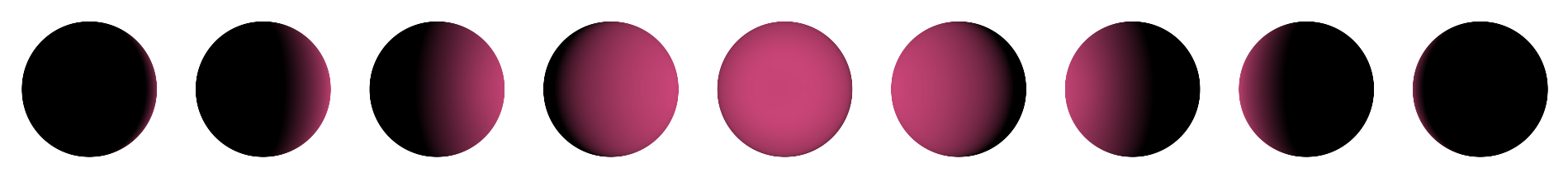The most obvious difference is at full phase, where the body appears to be super-Lambertian: more light is scattered back to the observer than you’d get in the isotropic case. This is mainly due to the behavior at the limb. For a Lambertian surface, the surface normal for points along the limb is nearly parallel to the light rays, so those regions jut don’t get illuminated much. But if the surface is very rough, there will be lots of facets near the limb that are oriented more perpendicular to the incident light, therefore reflecting much more light back to the observer at full phase. There are differences at other phases, too, which we can see more clearly by looking at the phase curve of the two bodies:

:

phase = np.linspace(0, 1, 1000)
xs = np.sin(2 * np.pi * phase)
zs = -np.cos(2 * np.pi * phase)

for roughness in [0, 30]:
map.roughness = roughness
plt.plot(
phase,
map.flux(xs=xs, zs=zs),
label=r"$\sigma = {:.0f}^\circ$".format(roughness),
)
plt.legend()
plt.xlabel("phase")
plt.ylabel("flux");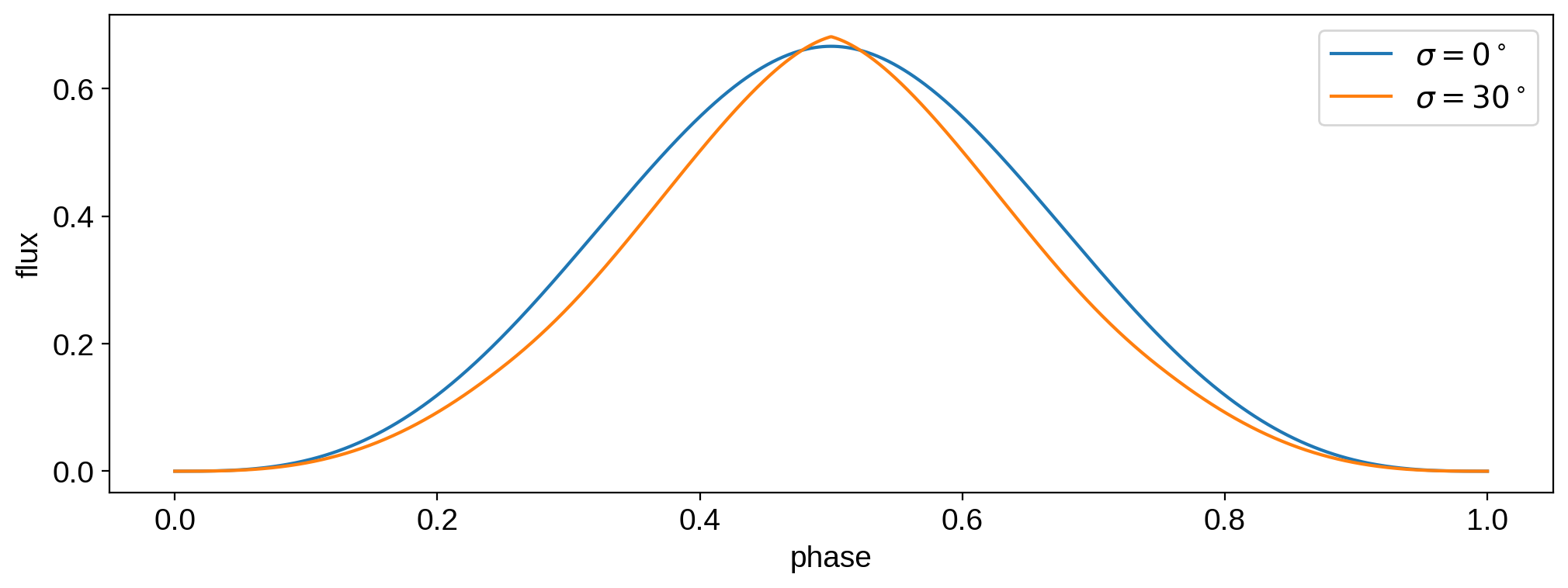The super-Lambertian nature of the body is clear right at full phase (0.5 in this figure). But at all other phases, the surface roughness causes less light to be reflected back to the observer.

The implementation of the Oren and Nayar model in starry is described in detail in this paper. It is a polynomial approximation to the actual Oren and Nayar model, but it’s computed analytically and works for maps with non-uniform albedo distributions and even during occultations.

Note

Another form of non-Lambertian scattering that is relevant to exoplanets – particularly terrestrial ones – is specular reflection. For planets with oceans like the Earth, this is known as glint. It’s tricky to model this in starry, since the glint spot is usually very small and hard to capture with spherical harmonics. But we’re currently working on ways to incorporate it, so stay tuned!

## Occultations¶

So far we’ve only discussed phase curves, but starry can compute occultation light curves in reflected light, too. These work just like in the emitted light case: the user provides the occultor Cartesian positions, xo, yo, and (optionally) zo, as well as its radius ro, all in unitss of the body’s radius.

Let’s compute a mock light curve of the Moon occulting the Earth:

:

# The Earth at l = 15
map = starry.Map(15, reflected=True)

# Set up the plot
nim = 8
npts = 100
nptsnum = 10
res = 300
fig = plt.figure(figsize=(12, 5))
ax_im = [plt.subplot2grid((4, nim), (0, n)) for n in range(nim)]
ax_lc = plt.subplot2grid((4, nim), (1, 0), colspan=nim, rowspan=3)

# Moon occultation params
ro = 0.273
yo = np.linspace(-0.5, 0.5, npts)
yonum = np.linspace(-0.5, 0.5, nptsnum)
xo = np.linspace(-1.5, 1.5, npts)
xonum = np.linspace(-1.5, 1.5, nptsnum)

# Say the occultation occurs over ~1 radian of the Earth's rotation
# That's equal to 24 / (2 * pi) hours
time = np.linspace(0, 24 / (2 * np.pi), npts)
timenum = np.linspace(0, 24 / (2 * np.pi), nptsnum)
theta0 = 0
theta = np.linspace(theta0, theta0 + 180.0 / np.pi, npts, endpoint=True)
thetanum = np.linspace(theta0, theta0 + 180.0 / np.pi, nptsnum, endpoint=True)

# Position of the illumination source (the sun).
# We'll assume it's constant for simplicity
xs = -1.0 * np.ones_like(time)
ys = 0.3 * np.ones_like(time)
zs = 1.0 * np.ones_like(time)

# Compute the flux
F = map.flux(theta=theta, xo=xo, yo=yo, ro=ro, xs=xs, ys=ys, zs=zs)
ax_lc.plot(time, F / np.max(F), "C0-", label="analytic")

# Plot the earth images
for n in range(nim):

# Show the image
i = int(np.linspace(0, npts - 1, nim)[n])
map.show(
ax=ax_im[n],
cmap=cmap,
xs=xs[i],
ys=ys[i],
zs=zs[i],
theta=theta[i],
res=res,
grid=False,
)

# Outline
x = np.linspace(-1, 1, 1000)
y = np.sqrt(1 - x ** 2)
f = 0.98
ax_im[n].plot(f * x, f * y, "k-", lw=0.5, zorder=0)
ax_im[n].plot(f * x, -f * y, "k-", lw=0.5, zorder=0)

# Occultor
x = np.linspace(xo[i] - ro + 1e-5, xo[i] + ro - 1e-5, res)
y = np.sqrt(ro ** 2 - (x - xo[i]) ** 2)
ax_im[n].fill_between(
x, yo[i] - y, yo[i] + y, fc="#aaaaaa", zorder=1, clip_on=False, ec="k", lw=0.5,
)
ax_im[n].axis("off")
ax_im[n].set_xlim(-1.05, 1.05)
ax_im[n].set_ylim(-1.05, 1.05)

# Appearance
ax_lc.set_xlabel("time [hours]", fontsize=16)
ax_lc.set_ylabel("normalized flux", fontsize=16)
for tick in ax_lc.get_xticklabels() + ax_lc.get_yticklabels():
tick.set_fontsize(14)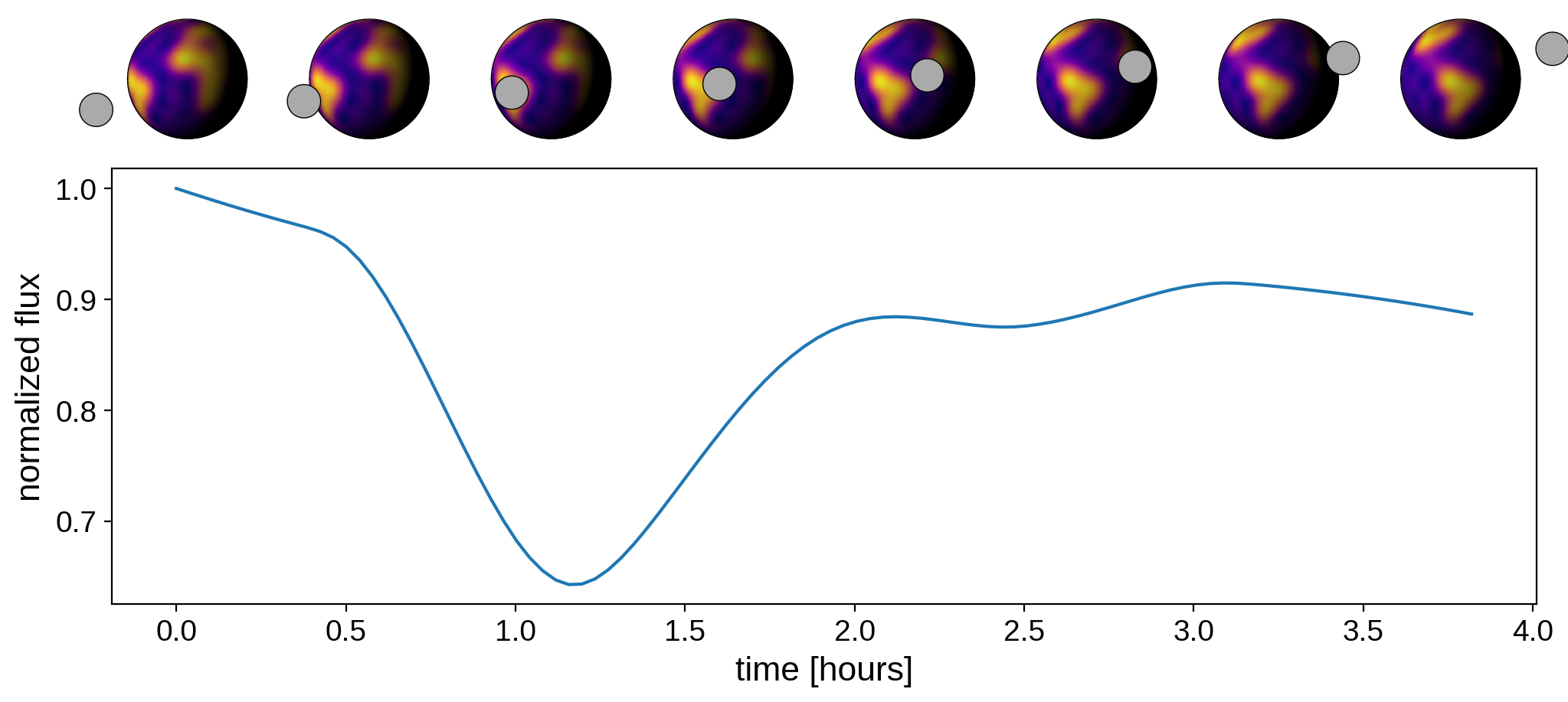## Modeling exoplanet systems¶

Finally, we can also model exoplanet systems using the starry.System class, just like in emitted light.

Here’s the phase curve of the Earth over one year in orbit around the Sun, seen from an orientation where the Earth’s orbital inclination is $$60^\circ$$:

:

sun = starry.Primary(starry.Map(), r=1, length_unit=u.Rsun)
earth = starry.Secondary(
map, porb=365.0, prot=1.0, m=0.0, inc=60, r=1, length_unit=u.earthRad
)
earth.map.inc = earth.inc = 60
earth.map.amp = 0.4
sys = starry.System(sun, earth)

t = np.linspace(0, 365.0, 1000)
plt.figure(figsize=(12, 5))
plt.plot(t, 1e9 * (sys.flux(t) - 1))
plt.xlabel("time [days]")
plt.ylabel("reflected planet flux [ppb]");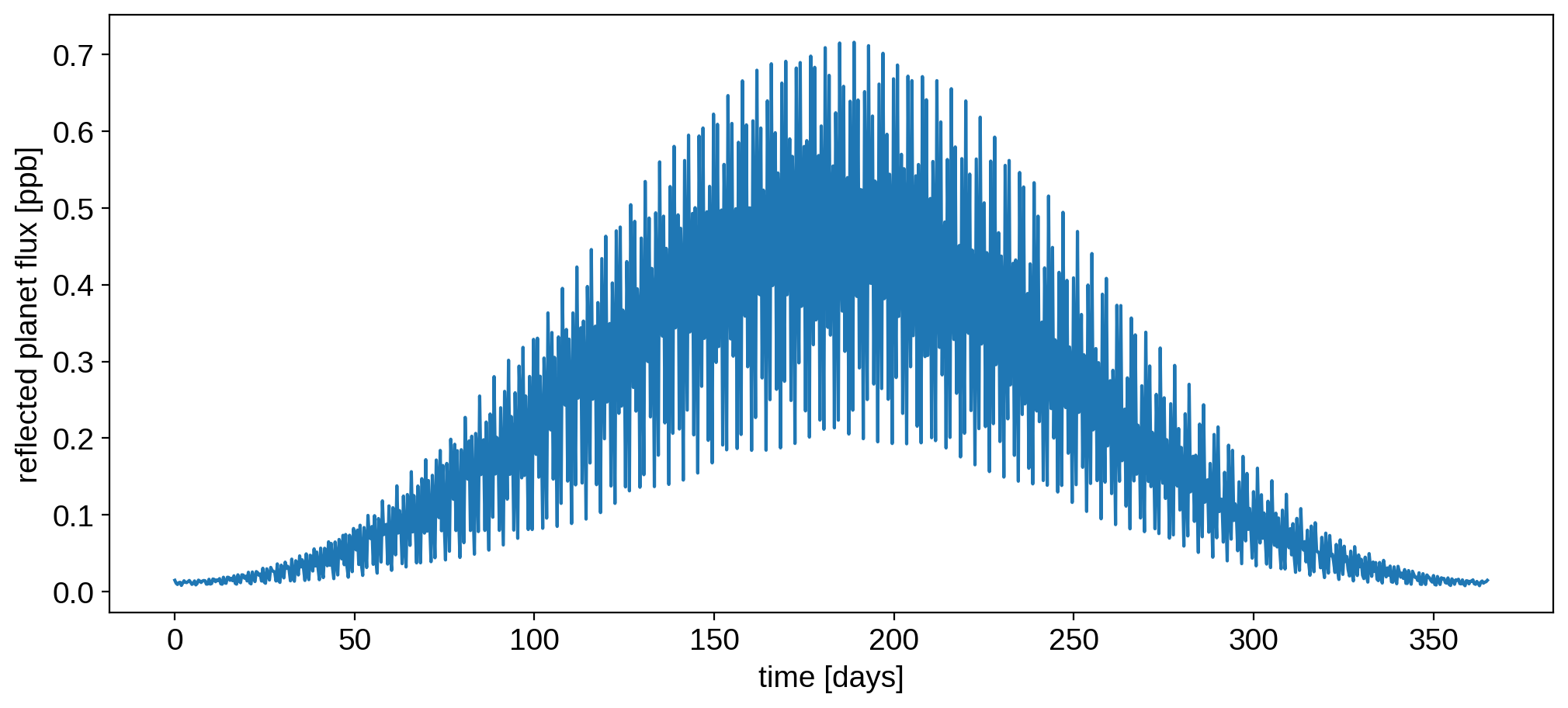The signal for the Earth is super tiny – it’s less than one part per billion ($$10^{-9}$$), but future coronagraph-equipped high-contrast space telescopes like LUVOIR may be able to detect this!

If we were to view the system edge-on, we’ll see the transit and the secondary eclipse. Let’s zoom in on the region near full phase to look at the secondary eclipse:

:

earth.map.inc = earth.inc = 90

t = np.linspace(365.0 / 2 - 1, 365.0 / 2 + 1, 1000)
plt.figure(figsize=(12, 5))
plt.plot(t, 1e9 * (sys.flux(t) - 1))

plt.xlabel("time [days]")
plt.ylabel("reflected planet flux [ppb]");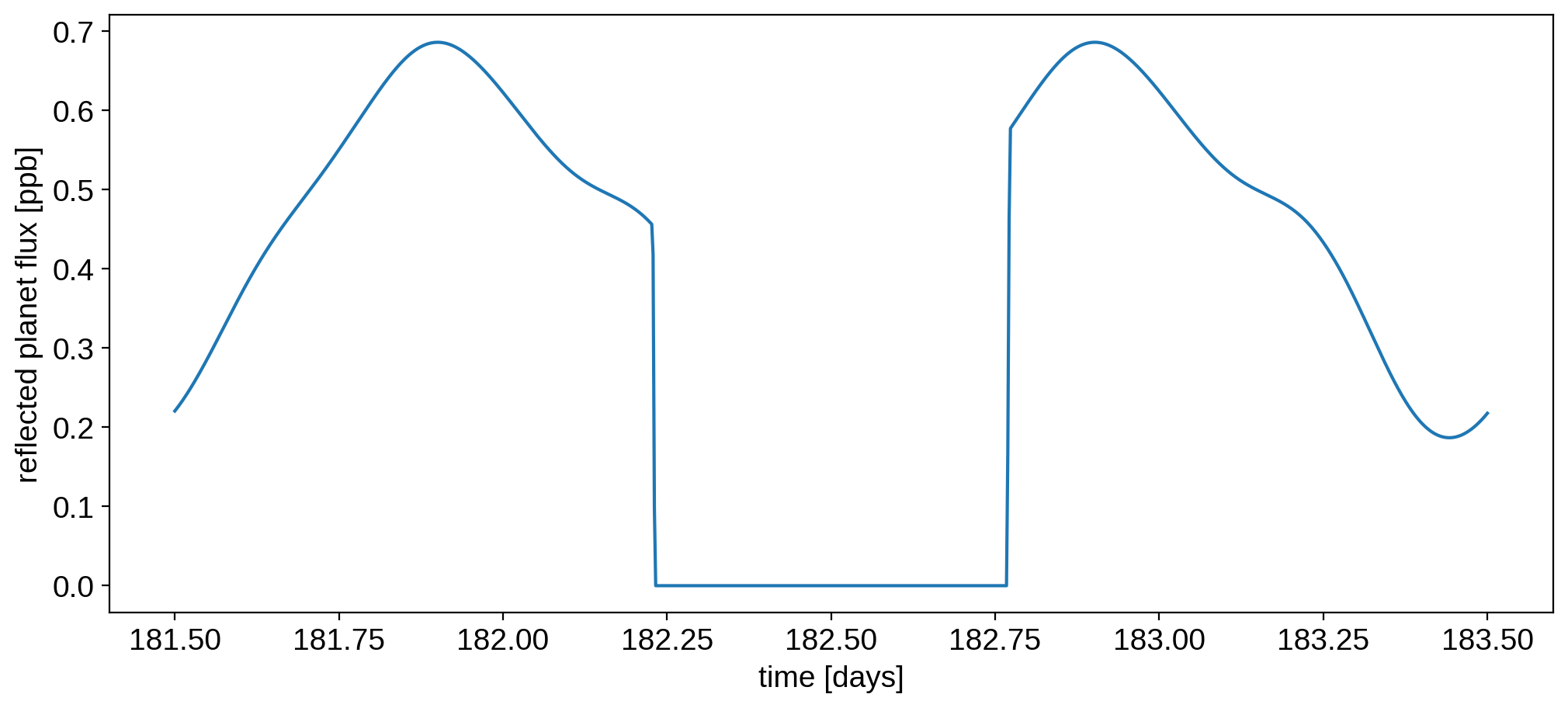We can also zoom in right at ingress and egress:

:

fig, ax = plt.subplots(1, 2, figsize=(12, 5), sharey=True)

# Ingress
t = np.linspace(182.225, 182.235, 1000)
ax.plot(t - t, 1e9 * (sys.flux(t) - 1))

# Egress
t = np.linspace(182.765, 182.775, 1000)
ax.plot(t - t, 1e9 * (sys.flux(t) - 1))

ax.set_xlabel("time [days]")
ax.set_xlabel("time [days]")
ax.set_title("ingress")
ax.set_title("egress")
ax.set_ylabel("reflected planet flux [ppb]");